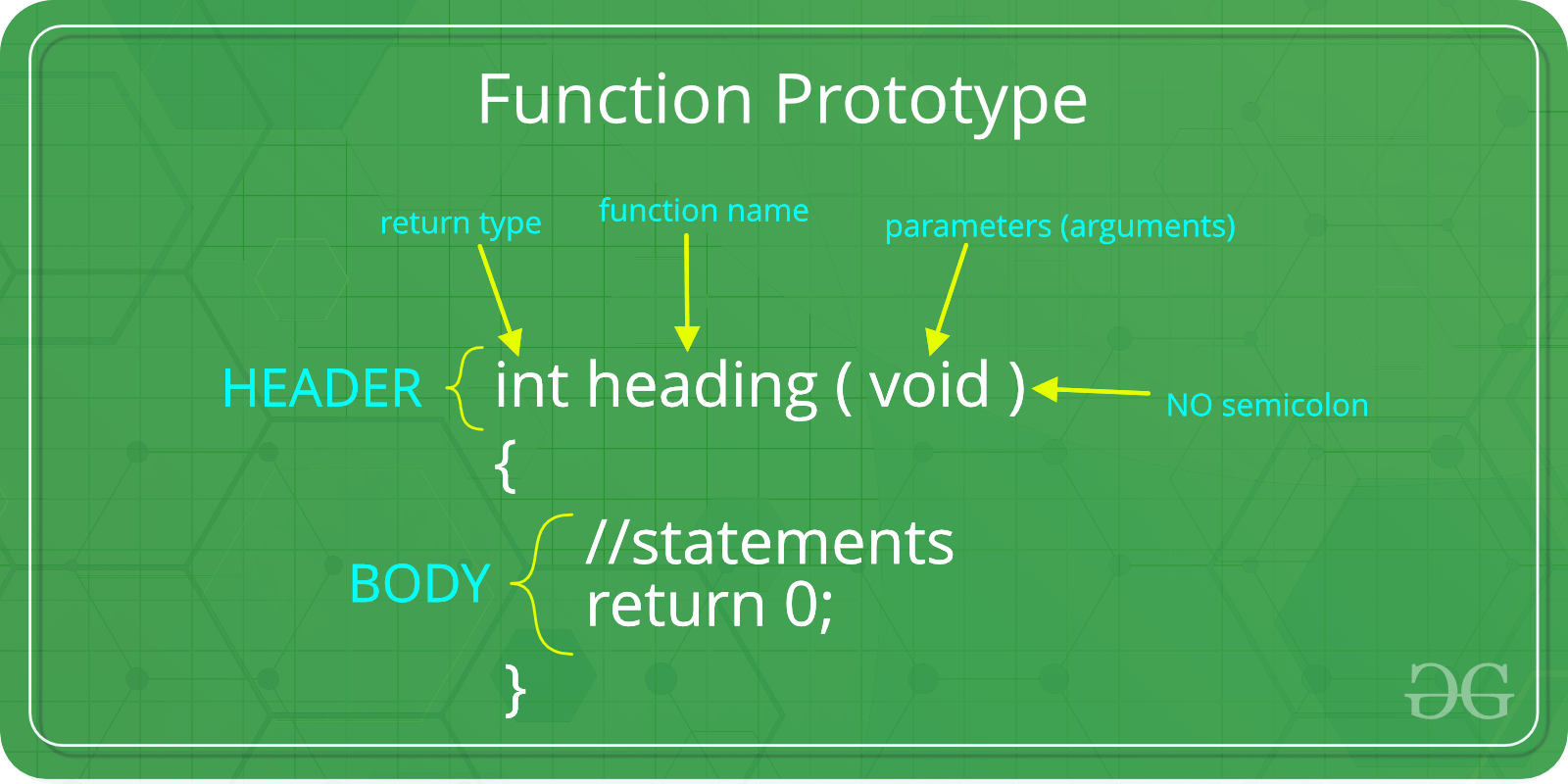Open In App

# C Function Arguments and Function Return Values

Prerequisite: Functions in C

A function in C can be called either with arguments or without arguments. These functions may or may not return values to the calling functions. All C functions can be called either with arguments or without arguments in a C program. Also, they may or may not return any values. Hence the function prototype of a function in C is as below:## Call by Value

Call by value in C is where in the arguments we pass value and that value can be used in function for performing the operation. Values passed in the function are stored in temporary memory so the changes performed in the function don’t affect the actual value of the variable passed.

Example:

## C

 `// C Program to implement``// Call by value``#include `` ` `// Call by value``int` `sum(``int` `x, ``int` `y)``{``    ``int` `c;``    ``c = x + y;`` ` `    ``// Integer value retured``    ``return` `c;``}`` ` `// Driver Code``int` `main()``{``    ``// Integer Declared``    ``int` `a = 3, b = 2;`` ` `    ``// Function Called``    ``int` `c = sum(a, b);``    ``printf``(``"Sum of %d and %d : %d"``, a, b, c);`` ` `    ``return` `0;``}`

Output

`Sum of 3 and 2 : 5`

## Call by Reference

Call by reference is the method in C where we call the function with the passing address as arguments. We pass the address of the memory blocks which can be further stored in a pointer variable that can be used in the function. Now, changes performed in the values inside the function can be directly reflected in the main memory.

Example:

## C

 `// C Program to implement``// Call by reference``#include `` ` `// Call by reference``void` `swap(``int``* x, ``int``* y)``{``    ``int` `temp = *x;``    ``*x = *y;``    ``*y = temp;``}`` ` `// Driver Code``int` `main()``{``    ``// Declaring Integer``    ``int` `x = 1, y = 5;``    ``printf``(``"Before Swapping: x:%d , y:%d\n"``, x, y);`` ` `    ``// Calling the function``    ``swap(&x, &y);``    ``printf``(``"After Swapping: x:%d , y:%d\n"``, x, y);`` ` `    ``return` `0;``}`

Output

```Before Swapping: x:1 , y:5
After Swapping: x:5 , y:1```

## Types of Function According to Arguments and Return Value

Functions can be differentiated into 4 types according to the arguments passed and value returns these are:

1. Function with arguments and return value
2. Function with arguments and no return value
3. Function with no arguments and with return value
4. Function with no arguments and no return value

### 1. Function with arguments and return value

Syntax:

```Function declaration : int function ( int );
Function call : function( x );
Function definition:
int function( int x )
{
statements;
return x;
}       ```

Example:

## C

 `// C code for function with arguments``// and return value``#include ``#include `` ` `int` `function(``int``, ``int``[]);`` ` `int` `main()``{``    ``int` `i, a = 20;``    ``int` `arr = { 10, 20, 30, 40, 50 };`` ` `    ``a = function(a, &arr);`` ` `    ``printf``(``"value of a is %d\n"``, a);``    ``for` `(i = 0; i < 5; i++) {``        ``printf``(``"value of arr[%d] is %d\n"``, i, arr[i]);``    ``}`` ` `    ``return` `0;``}`` ` `int` `function(``int` `a, ``int``* arr)``{``    ``int` `i;``    ``a = a + 20;`` ` `    ``arr = arr + 50;``    ``arr = arr + 50;``    ``arr = arr + 50;``    ``arr = arr + 50;``    ``arr = arr + 50;`` ` `    ``return` `a;``}`

Output

```value of a is 40
value of arr is 60
value of arr is 70
value of arr is 80
value of arr is 90
value of arr is 100```

### 2. Function with arguments but no return value

When a function has arguments, it receives any data from the calling function but it returns no values. These are void functions with no return values.

Syntax:

```Function declaration : void function ( int );
Function call : function( x );
Function definition:
void function( int x )
{
statements;
}
```

Example:

## C

 `// C code for function``// with argument but no return value``#include `` ` `void` `function(``int``, ``int``[], ``char``[]);`` ` `int` `main()``{``    ``int` `a = 20;``    ``int` `ar = { 10, 20, 30, 40, 50 };``   ` `    ``char` `str = ``"geeksforgeeks"``;``       ` `      ``// function call``    ``function(a, &ar, &str);``   ` `    ``return` `0;``}`` ` `void` `function(``int` `a, ``int``* ar, ``char``* str)``{``    ``int` `i;``    ``printf``(``"value of a is %d\n\n"``, a);``   ` `    ``for` `(i = 0; i < 5; i++) {``        ``printf``(``"value of ar[%d] is %d\n"``, i, ar[i]);``    ``}``   ` `    ``printf``(``"\nvalue of str is %s\n"``, str);``}`

Output

```value of a is 20

value of ar is 10
value of ar is 20
value of ar is 30
value of ar is 40
value of ar is 50

value of str is geeksforgeeks```

### 3. Function with no argument and no return value

When a function has no arguments, it does not receive any data from the calling function. Similarly, when it does not return a value, the calling function does not receive any data from the called function.

Syntax:

```Function declaration : void function();
Function call : function();
Function definition :
void function()
{
statements;
}
```

Example:

## C

 `// C code for function with no``// arguments and no return value``#include `` ` `void` `value(``void``);`` ` `void` `main() { ``  ``value(); ``}`` ` `void` `value(``void``)``{``    ``float` `year = 1, period = 5, amount = 5000,``          ``inrate = 0.12;``    ``float` `sum;``    ``sum = amount;``    ``while` `(year <= period) {``        ``sum = sum * (1 + inrate);``        ``year = year + 1;``    ``}``    ``printf``(``" The total amount is :%f"``, sum);``}`

Output

` The total amount is :8811.708984`

### 4. Function with no arguments but returns a value

There could be occasions where we may need to design functions that may not take any arguments but returns a value to the calling function. An example of this is getchar function which has no parameters but it returns an integer and integer-type data that represents a character.

Syntax:

```Function declaration : int function();
Function call : function();
Function definition :
int function()
{
statements;
return x;
}
```

Example:

## C

 `// C code for function with no arguments``// but have return value``#include ``#include `` ` `int` `sum();`` ` `int` `main()``{``    ``int` `num;``    ``num = sum();``    ``printf``(``"Sum of two given values = %d"``, num);``    ``return` `0;``}`` ` `int` `sum()``{``    ``int` `a = 50, b = 80, sum;``    ``sum = ``sqrt``(a) + ``sqrt``(b);``    ``return` `sum;``}`

Output

`Sum of two given values = 16`